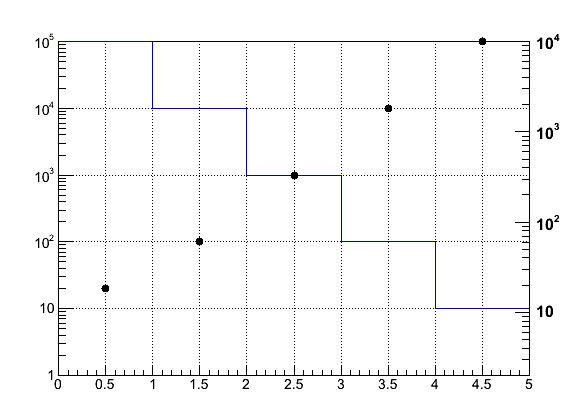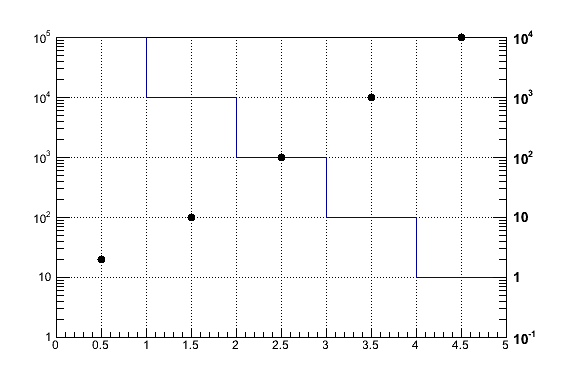# How to overlay multiple histograms with 2 axes and log scale

Hi,

I’m trying to overlay two histograms with two y-axes in a manner similar to http://root.cern.ch/root/html/tutorials/hist/twoscales.C.html. My problem is how to get log scales to work using this method. When I turn on log scale the overlayed histograms points and the 2nd y-axis no longer properly correspond.

Here is a simple macro to show the effect:

``````TCanvas *c1;
TH1D *h1, *h2;
TGaxis *ax1;

void t3()
{
const Int_t nbinX = 5;
Double_t hX[nbinX + 1] = {0., 1., 2., 3., 4., 5.};
Double_t h1Y[nbinX] = {100000., 10000., 1000., 100., 10.};

// first histogram
h1 = new TH1D("h1", "h1", nbinX, hX);
h1->SetStats(kFALSE);
for (Int_t i = 1; i < nbinX + 1; i++)
h1->SetBinContent(i, h1Y[i - 1]);

// second histogram to overlay
Double_t h2Y[nbinX] = {2, 10, 100, 1000, 10000};
h2 = new TH1D("h2", "h2", nbinX, hX);
h2->SetStats(kFALSE);
h2->SetMarkerStyle(kFullCircle);
for (Int_t i = 1; i < nbinX + 1; i++)
h2->SetBinContent(i, h2Y[i - 1]);

c1 = new TCanvas("c1", "c1");
h1->Draw();
c1->Update();

Double_t h2min = h2->GetMinimum();

Float_t rightmax = 1.1*h2->GetMaximum();
h2->Scale(scale);
h2->Draw("p same");

h2min, rightmax, 510, "+LG");
ax1->Draw();

c1->SetLogy();
}``````

The solid histogram and y-axis on the left (h1) are correct, the points and the y-axis on the right (h2) are not correct. The points should be at 2, 10, 100, 1000, 10000, but they are slightly off.

How does one properly do this?

Yes, that example is based on the assumption we work in linear scale.
I am now trying to make it work in log-scale.
In particular the way the “scale” value is computed is wrong. It should be 10. It is not.
print it to see …

``````TCanvas *c1;
TH1D *h1, *h2;
TGaxis *ax1;

void t3()
{
const Int_t nbinX = 5;
Double_t hX[nbinX + 1] = {0., 1., 2., 3., 4., 5.};
Double_t h1Y[nbinX] = {100000., 10000., 1000., 100., 10.};

// first histogram
h1 = new TH1D("h1", "h1", nbinX, hX);
h1->SetStats(kFALSE);
for (Int_t i = 1; i < nbinX + 1; i++) h1->SetBinContent(i, h1Y[i - 1]);

// second histogram to overlay
Double_t h2Y[nbinX] = {2, 10, 100, 1000, 10000};
h2 = new TH1D("h2", "h2", nbinX, hX);
h2->SetStats(kFALSE);
h2->SetMarkerStyle(kFullCircle);
for (Int_t i = 1; i < nbinX + 1; i++) h2->SetBinContent(i, h2Y[i - 1]);

c1 = new TCanvas("c1", "c1");
c1->SetLogy();
c1->DrawFrame(0.,1.,5.,100000.);
h1->Draw("same");
c1->Update();

Double_t h1min = h1->GetMinimum();
Double_t h1max = h1->GetMaximum();
Double_t h2min = h2->GetMinimum();
Double_t h2max = h2->GetMaximum();

Double_t scale = h1max/h2max;
printf("scale = %g\n",scale);
h2->Scale(scale);
printf("scale=%g pmax = %g h2max = %g\n",
scale,pymax,h2max);

h2->Draw("p same");

h2min, h2max, 510, "+LG");
ax1->Draw();

c1->SetGridy(1);
}
``````

Thank you for responding. Your code gives me the exact same result though.ah yes sorry.
do:

``````ax1 = new TGaxis(gPad->GetUxmax(), pymin,
0.1, h2max, 510, "+LG");
``````

That works for me now.

OK, so if I understand correctly, you have to match the scale variable to h1max/h2max. This fixes the shift I’d see in the overlayed histogram when setting the canvas to log scale (c1->SetLogy()), I believe. And you need to match the same number of decades in the overlayed axis range. The real data I’m working with, of course, doesn’t have such simple values to work with.For scaling of the overlayed axis then for arbitrary data, I need to know then the displayed range of h1’s y-axis. If I fixed the upper bound of h2’s axis to h2max and then compute the lower bound I need to do something like:

``````   // displayed range of h1's axis after doing c1->SetLogy()
Double_t y1max = h1->GetYaxis()->GetXmax();
Double_t y1min = h1->GetYaxis()->GetXmin();

// displayed range of h2's axis drawn in log scale (using option "G")
Double_t y2max = h2max;
Double_t y2min = TMath::Power(10., TMath::Log10(y2max) -
(TMath::Log10(y1max) - TMath::Log10(y1min)));

std::cout << "h1 axis: [" << y1max << " : " << y1min << "]" << std::endl;
std::cout << "h2 axis: [" << y2max << " : " << y2min << "]" << std::endl;

y2min, y2max, 510, "+LG");``````

The problem is that GetYaxis()->GetXmax() and GetYaxis()->GetXmin() don’t provide valid results (I get 1 and 0, respectively). Using GetUymin and GetUymax also return wrong results: 0 and 5 (I also get the same exact values for GetUxmin and GetUxmax, this seems like an error).

How then do I get the displayed range for an axis?

I do this all the time. I ask the question, then come up with the (partial) answer.

I see that you used DrawFrame, which I wasn’t familiar with, but you set the h1 axis range from 1 - 10^5. GetUymin and GetUymax, I think return the log values when in log scale, so getting 0 and 5 are correct. I guess I can get the y1min and y1max since we are setting it explicitly by hand using GetFrame. Or if I really wanted to read it out, I could use GetFrame()->GetY1(), GetFrame()->GetY2().

I’ll try applying this to my real data and see how it all works.

Thanks for the assistance.

Here’s my “final” test version of how to accomplish this. I’m posting it for others that may need this solution in the future.

This provides margins on the histogram and determines the proper length of the h2 axis by calculating it (matching decades for h1 and h2). If you see any potential problems with it, feel free to comment.

``````TCanvas *c1;
TH1D *h1, *h2;
TGaxis *ax1;

void t4()
{
const Int_t nbinX = 5;

// margins on the histogram min/max applied to rescaled axes. for log scale
// 50% or more is good.
const Double_t margin = 0.5;

Double_t hX[nbinX + 1] = {0., 1., 2., 3., 4., 5.};
Double_t h1Y[nbinX] = {100000., 10000., 1000., 100., 10.};

// first histogram
h1 = new TH1D("h1", "h1", nbinX, hX);
h1->SetStats(kFALSE);
for (Int_t i = 1; i < nbinX + 1; i++)
h1->SetBinContent(i, h1Y[i - 1]);

Double_t y1max = (1. + margin)*h1->GetMaximum();
Double_t y1min = (1. - margin)*h1->GetMinimum();

// second histogram to overlay
Double_t h2Y[nbinX] = {2., 10., 100., 1000., 10000.};
h2 = new TH1D("h2", "h2", nbinX, hX);
h2->SetStats(kFALSE);
h2->SetMarkerStyle(kFullCircle);
for (Int_t i = 1; i < nbinX + 1; i++)
h2->SetBinContent(i, h2Y[i - 1]);

c1 = new TCanvas("c1", "c1");
c1->SetLogy();
c1->DrawFrame(0., y1min, 5., y1max);
h1->Draw("same");
c1->Update();

Double_t h1min = h1->GetMinimum();
Double_t h1max = h1->GetMaximum();
Double_t h2min = h2->GetMinimum();
Double_t h2max = h2->GetMaximum();

Double_t scale = h1max/h2max;
printf("scale = %g\n",scale);
h2->Scale(scale);
printf("scale=%g pmax = %g h2min = %g h2max = %g\n",
scale,pymax,h2min,h2max);

h2->Draw("p same");

// when choosing the 2nd y-axis range, "fix" the upper bound, then solve
// for the lower bound so that the number of decades matches the same
// number of decades used in the 1st y-axis range.
Double_t y2max = (1. + margin)*h2max;
Double_t y2min = TMath::Power(10., TMath::Log10(y2max) -
(TMath::Log10(y1max) - TMath::Log10(y1min)));

// some debug info
std::cout << "h1 axis: [" << y1min << " : " << y1max << "]" << std::endl;
std::cout << "h2 axis: [" << y2min << " : " << y2max << "]" << std::endl;
std::cout << "h1 decades: " << TMath::Log10(y1max) - TMath::Log10(y1min) <<
std::endl;
std::cout << "h2 decades: " << TMath::Log10(y2max) - TMath::Log10(y2min) <<
std::endl;